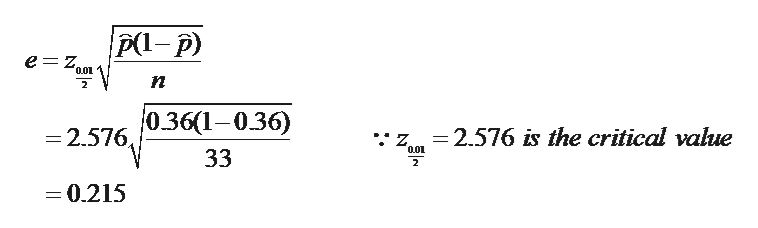# #12    Compute the following problem using the estimation of proportions tab in Excel. Write your answer in inequality notation, in the p+- e notation and graph the interval.x= 12    n= 33     c-level: 0.99p hat: __________

Question
1 views

#12    Compute the following problem using the estimation of proportions tab in Excel. Write your answer in inequality notation, in the p+- e notation and graph the interval.

x= 12    n= 33     c-level: 0.99

p hat: __________

check_circle

Step 1

Solution:

We are given:

Step 2

We have to find the estimate of the population proportion.

We know that:

Step 3

To find the 99% confidence interval estimate for the population proportion, we have to find the m...help_outlineImage TranscriptioncloseP- p) e Z, 0.01 п о361-036) =2.576 is the critical value -2.576, 0.01 33 2 = 0.215 fullscreen

### Want to see the full answer?

See Solution

#### Want to see this answer and more?

Solutions are written by subject experts who are available 24/7. Questions are typically answered within 1 hour.*

See Solution
*Response times may vary by subject and question.
Tagged in
MathStatistics

### Hypothesis Testing